Home Blog Blogs Modulator Bandwidth & Bitrate

# Modulator Bandwidth & Bitrate

07.06.2023What is the relationship between the maximum output bit rate of the modulator and the bandwidth, symbol rate, and constellation?

1. What is modulation bandwidth?

1.1 What is the formula for bandwidth in modulation?

1.2. Influencing factor: Roll-off

1.3 DVB-T/C/ATSC Modulator Bandwidth

1.4 Does a higher bitrate mean higher bandwidth?

2. What is the symbol rate in modulation?

2.1 What is a constellation modulation?

2.2 Images for modulation Constellation

2.3 Which kinds of constellations are used by different modulators standard currently on the market?

3. Modulation bit rate calculation

3.1 What's the relationship between symbol rate and maximum bit rate in modulation?

3.2 What is the formula for bit rate?

DVB-C max bit rate

DVB-S max bit rate

DVB-S2 max bit rate

DVB-T max bit rate

DMB-T max bit rate

ATSC-T max bit rate

ISDB-T max bit rate

3.3 How do you calculate the bit rate per channel?

3.4 How many modulators do we need to transmit 100 programs?

4. RF out

4.1 What is a digital modulator? (what does a modulator do?)

4.2 How to select the output bandwidth of one modulator?

### 1. What is modulation bandwidth?

Generally refers to the RF bandwidth of the modulated output spectrum. The unit is Hz. Commonly used are 8Mhz, 6Mhz, etc. DVB-S/DVB-S2 can be as high as 60.75 Mhz.

#### 1.1 What is the formula for bandwidth in modulation? How do you calculate bandwidth from the symbol rate?

Regardless of a single carrier or multi-carrier, the transmission bandwidth is directly related to the symbol rate relevant.

For single carrier:

Transmission Bandwidth = symbol rate * (1+ roll-off factor)

Take DVB-C as an example, the bandwidth as the picture shows: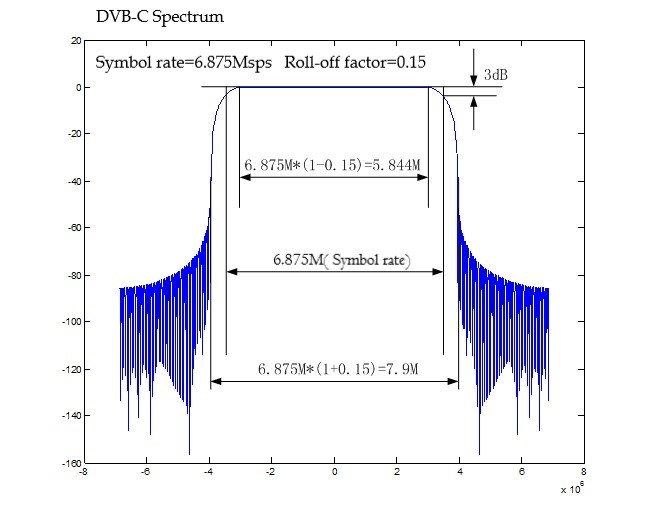For multi-carrier:

There is no specific formula, but the two are proportional

#### 1.2 Roll-off factor in modulation

Spectrum comparison of different roll-off coefficients

Blue-0.15 Red-0.20 Green-0.35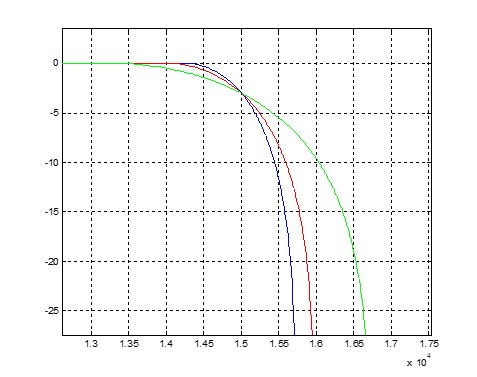Partial enlarged: the coincident point is the symbol rate point, attenuation 3db

#### 1.3 DVB-T/C/ATSC Modulator Bandwidth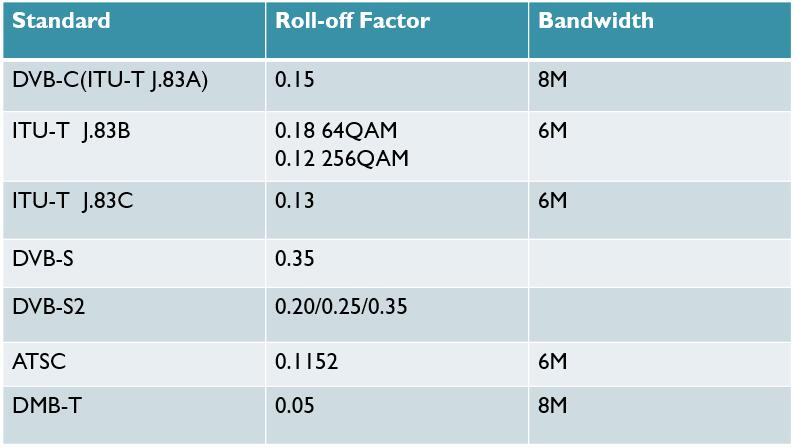Comparison of roll-off coefficients of different single-carrier standards

The larger the roll-off coefficient is, the smaller the symbol interference is when receiving, and the requirements for receiving are lower, but the transmission bandwidth increases.

The smaller the roll-off coefficient, the greater the symbol interference during reception, which requires high reception, but saves transmission bandwidth.

#### 1.4 Does a higher bitrate mean higher bandwidth?

In practice application, bandwidth depends on what modulation standard is used in your country and the rules on which bandwidth is allowed to use.

Take the DVB-T standard as an example, in which areas 8M is used, and in which areas 7M is used.

### 2. What is the symbol rate in modulation?

Symbol rate: specifically refers to the symbol rate in modulation, that is, the rate at which a constellation point on the constellation is transmitted. The unit is sps (symbols per second). The symbol rate is related to the maximum input code rate and RF bandwidth.

The symbol rate range supported by the modulator, taking a single-channel DVB-C modulator as an example, is 0.5~45M, commonly used as 6.875M or other values.

#### 2.1 What is a constellation modulation?

Constellation modulation is the art of encoding information not only in the symbols within a constellation but also by encoding information by selecting a constellation from a set of constellations that are switched from time to time.

#### 2.2 Diagrams for modulation constellation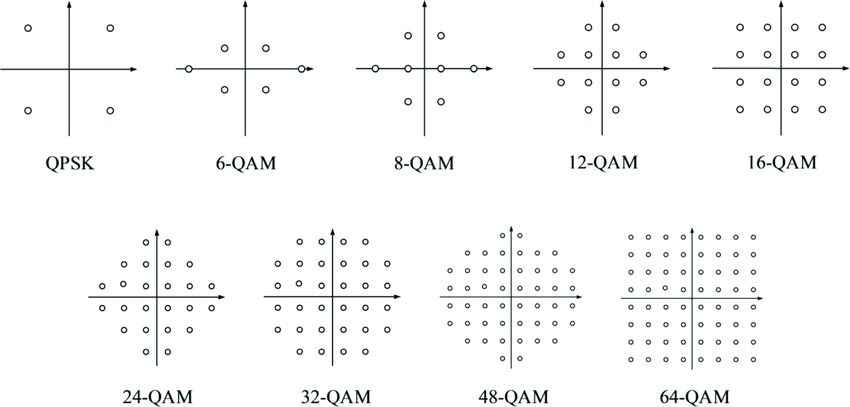#### 2.3 Which kinds of constellations are used by different modulator standards currently on the market?

DVB-C modulator constellations: 16, 32, 64, 128, 256QAM

DVB-T modulator constellations: QPSK, 16QAM, 64QAM

DVB-T2 modulator constellations: QPSK, 16QAM, 64QAM

ATSC-T  modulator constellations: 8VSB

ISDB-T modulator constellations: QPSK, 16QAM, 64QAM

DVB-S modulator constellations: QPSK

DVB-S2  modulator constellations: QPSK, 8PSK, 16APSK, 32APSK

### 3. Modulation bit rate

Bit rate: refers to the TS code rate, in bps (bits per second).

#### 3.1 What is the relationship between symbol rate and maximum bit rate in modulation?

For a single carrier, the maximum code rate is closely related to the symbol rate, FEC code rate, and constellation diagram, and the relationship is as follows:

Symbol rate ↑                 Maximum code rate ↑       Receive threshold →

FEC code rate ↑              Maximum code rate ↑       Receive threshold ↑

Constellation Level ↑     Maximum Bit Rate ↑          Reception Threshold ↑

For multi-carriers, the general symbol rate is fixed, and the rest of the relationship is the same as above. In addition, multi-carriers are also related to guard intervals, and the relationship is as follows:

Guard interval ↑             Maximum code rate ↓       Receive threshold →

#### 3.2 What is the formula for bit rate in modulation?

Under different modulator standards, the maximum code rate calculation formula:

DVB-C(<=38.14Mbps)

Maximum code rate = symbol rate * (188/204) * constellation BIT

6.875M 64QAM 6.875M*(188/204)*6=38.14Mbps

DVB-S

Maximum code rate = symbol rate * (188/204) * FEC_RATE * 2 (constellation BIT)

DVB-S2

Max_TS_bitrate =Symbol_rate*spectral_efficiency

The calculation is more troublesome, see the following table: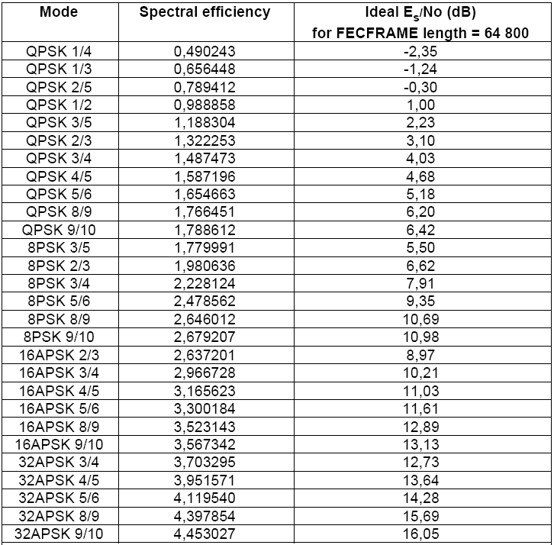DVB-T2

Maximum code rate=52Mbps

DVB-T(<=31Mbps);

Maximum code rate=64M/7(symbol rate)* (188/204)*FEC_RATE

*Constellation BIT*(1/(1+GI))

Table 1: Useful bitrate(Mbit/s) for all combinations of guard interval, constellation, and code rate for non-hierarchical systems for 6MHz channels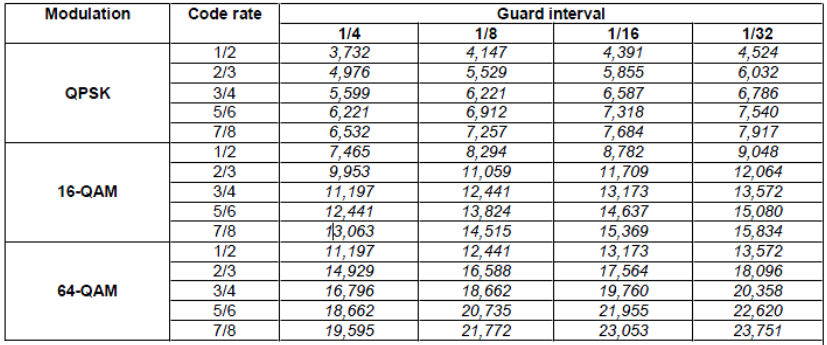Table1: DVB-T 6M Bandwidth Maximum Bit rate

NOTE: Figures in italics are approximate values.

For the hierarchical schemes, the useful bite rates can be obtained from Table 1 as follows:

HP stream: figures from QPSK columns.

LP stream: 16-QAM: figures from QPSK columns.

LP stream, 16-QAM: figures from 16-QAM columns

Table 2: Useful bitrate(Mbit/s) for all combinations of guard interval, constellation, and code rate for non-hierarchical systems for 7MHz channels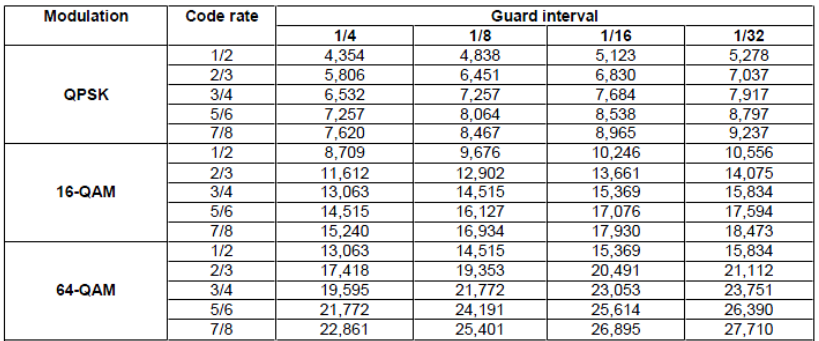Table 2: DVB-T 7M Bandwidth Maximum Bit rate

NOTE: Figures in italics are approximate values.

For the hierarchical schemes, the useful bite rates can be obtained from Table 2 as follows:

HP stream: figures from QPSK columns.

LP stream: 16-QAM: figures from QPSK columns.

LP stream, 64-QAM: figures from 16-QAM columns

Table 3: Useful bitrate (Mbit/s) for all combinations of guard interval, constellation, and code rate for non-hierarchical systems for 8MHz channelsTable 3: DVB-T 8M Bandwidth Maximum Bit rate

NOTE: Figures in italics are approximate values for 8MHz channels. Values for 6 MHz and 7 MHz channels are given in Annex E, Table 1 & Table 2.

For the hierarchical schemes, the useful bite rates can be obtained from Table 3 as follows:

HP stream: figures from QPSK columns.

LP stream: 16-QAM: figures from QPSK columns.

LP stream, 64-QAM: figures from 16-QAM columns

DMB-T

Maximum code rate=7.56M (symbol rate)*FEC_RATE*constellation BIT

ATSC-T

Maximum code rate=19.38Mbps

ISDB-T

Maximum code rate=23Mbps

ISDB-T(6MHz)

Table 4 shows the data rate per segment, while Table 4 presents the total data rate for all 13 segments.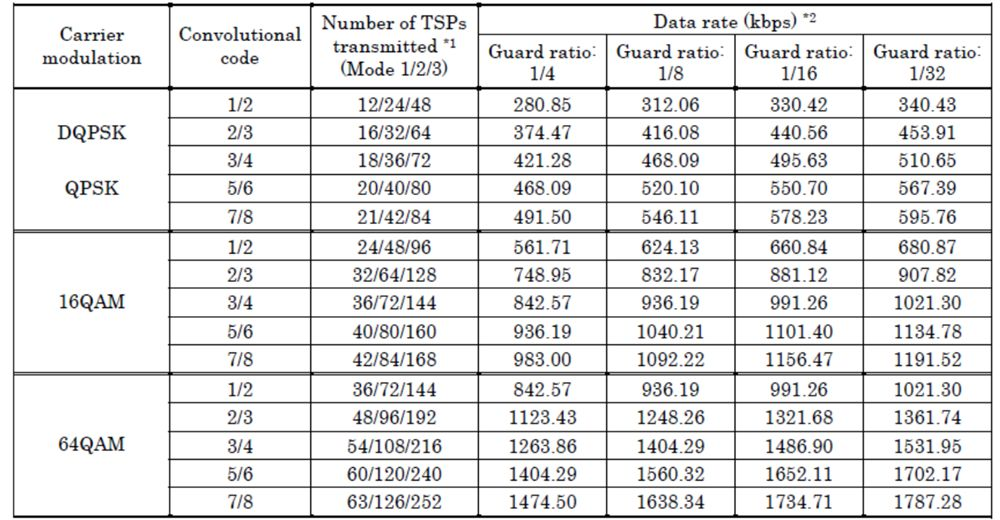*1: Represents the number of TSPs transmitted per frame

*2: Represents the data rate(bits) per segment for transmission parameters

Data rate(bits): TSPs transmitted 188(bytes/TSP)X8(bits/byte)x1/frame length

You need to check the limitation on the ISDB-T Multiplexer. For each Layer, please don’t multiplex the bit rate more than the number of segments multiplied by the data rate which is a lookup from Table 4.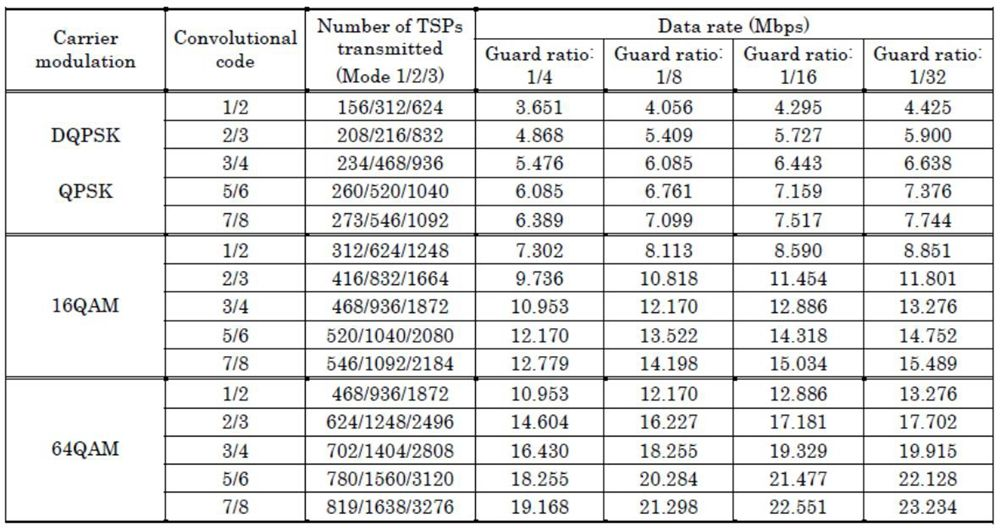*1: This table shows an example of the total data rate in which the same parameters are specified for all 13 segments. Note that the total data rate during hierarchical transmission varies depending on the hierarchical parameter configuration. In the case shown above, the data volume transmitted by all 13 segments is equal to the sum of all data volumes transmitted by these segments which can be determined based on Table 5.

For 7MHz( or 8MHz) bandwidth, please calculate it as 6MHz-result*7/6( or 6MHz_result*8/6).

#### 3.3 How do you calculate the bit rate per channel?

Go directly to the encoder to check the output bit rate of each program

Or through a tool: TSReader, Dektec, ASI analyzer, etc...

#### 3.4 How many modulators do we need to transmit 100 programs?

Each program bit rate * number of programs (100) / modulator output bit rate

### 4. RF Range

#### 4.1 What is a digital modulator? (what does a modulator do?)

The function of the modulator is to modulate the TS stream to DVBC/DVBT and other carriers and output it to the set-top box/TV.

#### 4.2 How to select the output bandwidth of one modulator?

Take a client's TV listings as an example:

John told us “RF carrier output needs to be configurable between DVB-C Frequency Range, preferred Frequency Range is 194 ~ 650 MHz.”, and gave us his programs table: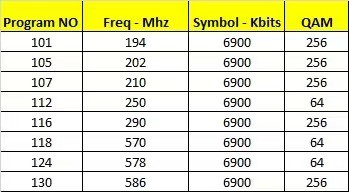A. From the table, we know we need a frequency range is:586-194=392MHz

B. We know the QAM RF output range is: 50~960MHz, while for one common modulator the max output is 192MHz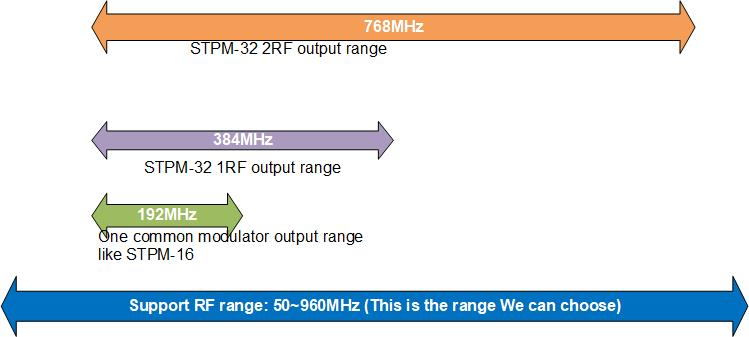STPM-32 RF*2 output model is suitable.